##### Physics I: 501 Practice Problems For Dummies (+ Free Online Practice)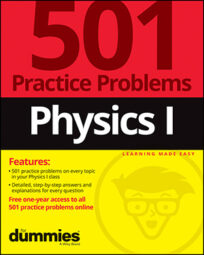Using physics, you can calculate the gravitational force that is exerted on one object by another object. For example, given the weight of, and distance between, two objects, you can calculate how large the force of gravity is between them.

Here are some practice questions that you can try.

## Practice questions

1. The gravitational force between objects A and B is 4 newtons. If the mass of B were one-half as large as it currently is while A's mass remains the same, how large is the gravitational force?

2. Calculate the force of gravity between two 3-kilogram ball bearings separated by a distance of 10 centimeters.

3. A 9,000-kilogram starship is pulled toward Planet X, a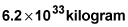behemoth with a radius of 65,000 kilometers. When the starship is 2,500 kilometers from the planet's surface, what is the starship's acceleration (providing that its engines are turned off)? Round your answer to two significant digits.

The following are the answers to the practice questions:

1. 2 N

The force of gravity exerted between objects is proportional to each object's mass. If B's mass is halved — with A's mass remaining unchanged — then the gravitational force between A and B is also halved: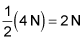2.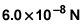Before you can substitute all the given values into the law of universal gravitation, you need to convert the distance between the ball bearings into meters to match the units in the gravitational constant, G: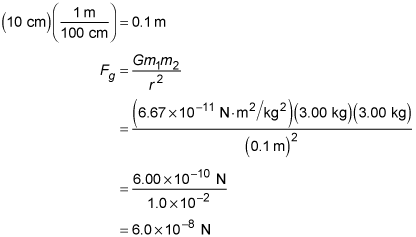3.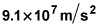With its engines off, the only force that the starship feels is the gravitational force attracting it to Planet X. Therefore, the net force on the starship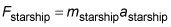must be equal to the force of gravity between the ship and the planet,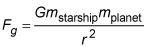r represents the distance between the centers of the two objects: The distance from the center of Planet X to its surface is 65,000 kilometers, and the distance from the surface to the starship is another 2,500 kilometers, making the total distance between the planet and the starship 67,500 kilometers — or, more importantly, given the units situation, 67,500,000 meters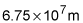Substituting all the data into the equations leaves you with: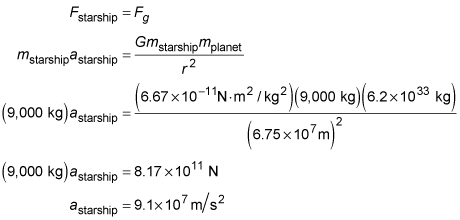(You can save yourself a little handwriting by noticing that because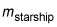appears on both sides of the equation in the second line, you can divide it from both sides to leave you with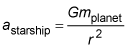an equation with the unknown already separated and only three substitutions to enter.)